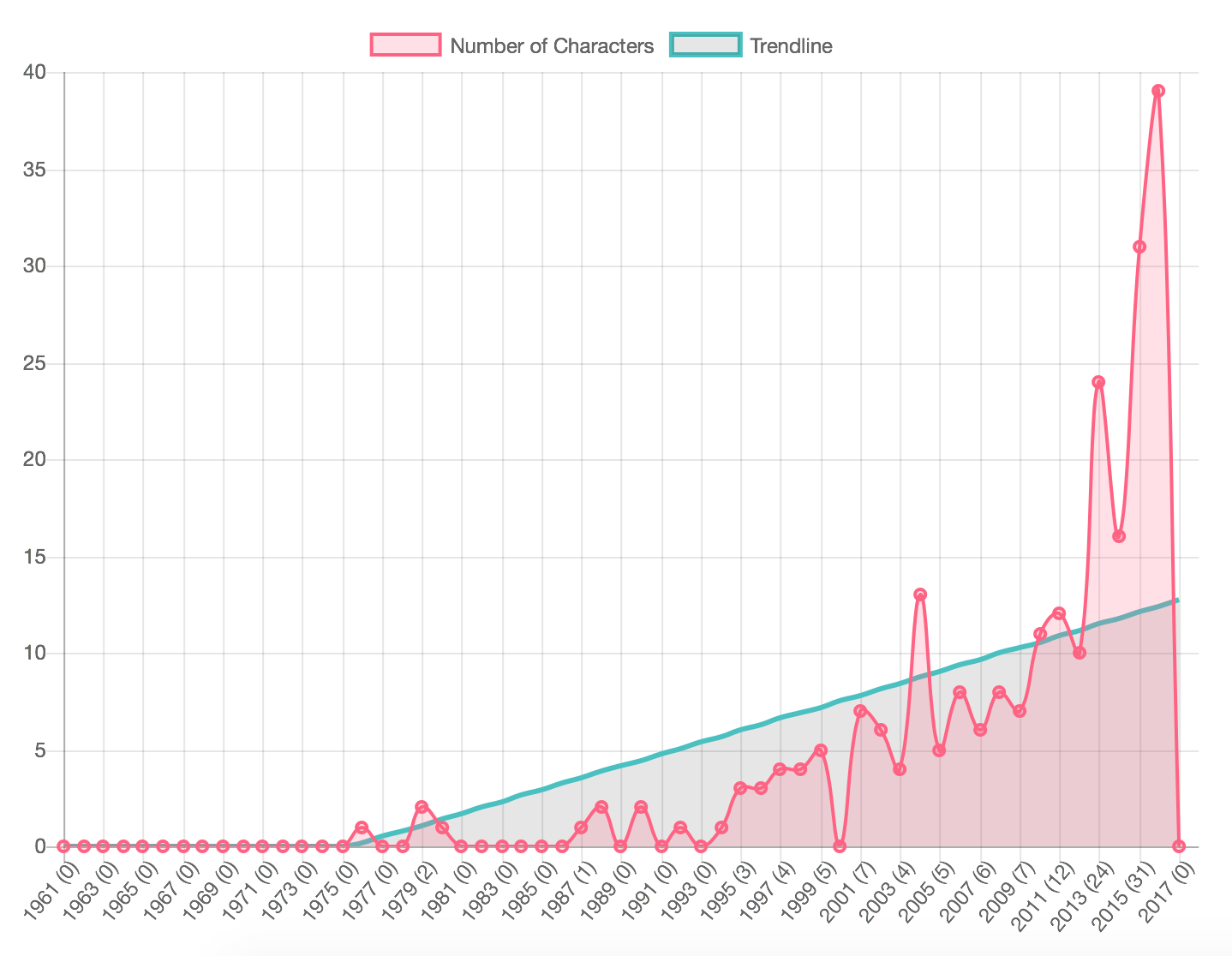[Machine learning] Linear regression in PHP

Linear regression in PHP

Using Google Docs to generate a trend line is easy. Enter the data and tell it to make a trend line. Using PHP to do this is a bit messier. I use Chart.js to generate my stats into pretty graphs, and while it gives me a lot of flexibility, it does not make the math easy. but gives us an insight for Linear regression in PHP.

I have an array of data for the years and the number of death per year. That’s the easy stuff. As of version 2.0 of Chart.js, you can stack charts, which lets me run two lines on top of each other like this:

 1 2 3 4 5 6 7 8 9 10 11 12 13 14 15 16 17 18 `var myChart = new Chart(ctx, {` `    ``type: 'bar',` `    ``data: {` `        ``labels: ['Item 1', 'Item 2', 'Item 3'],` `        ``datasets: [` `            ``{` `                ``type: 'line',` `                ``label: 'Line Number One',` `                ``data: [10, 20, 30],` `            ``},` `            ``{` `                ``type: 'line',` `                ``label: 'Line Number Two',` `                ``data: [30, 20, 10],` `            ``}` `        ``]` `    ``}` `});`

But. Having the data here doesn’t mean I know how to properly generate the trend. What I needed was the most basic formula solved: `y = x(slope) + intercept` and little more. Generating the slope an intercept are the annoying part.

For example, slope is `(NΣXY - (ΣX)(ΣY)) / (NΣX2 - (ΣX)2)` where,

• x and y are the variables.
• b = The slope of the regression line
• a = The intercept point of the regression line and the y axis.
• N = Number of values or elements
• X = First Score
• Y = Second Score
• ΣXY = Sum of the product of first and Second Scores
• ΣX = Sum of First Scores
• ΣY = Sum of Second Scores
• ΣX2 = Sum of square First Scores

 1 2 3 4 5 6 7 8 9 10 11 12 13 14 15 16 17 18 19 20 21 22 23 24 25 `function linear_regression( \$x, \$y ) {` `    ``\$n     = count(\$x);     // number of items in the array` `    ``\$x_sum = array_sum(\$x); // sum of all X values` `    ``\$y_sum = array_sum(\$y); // sum of all Y values` `    ``\$xx_sum = 0;` `    ``\$xy_sum = 0;` `    ``for(\$i = 0; \$i < \$n; \$i++) {` `        ``\$xy_sum += ( \$x[\$i]*\$y[\$i] );` `        ``\$xx_sum += ( \$x[\$i]*\$x[\$i] );` `    ``}` `    ``// Slope` `    ``\$slope = ( ( \$n * \$xy_sum ) - ( \$x_sum * \$y_sum ) ) / ( ( \$n * \$xx_sum ) - ( \$x_sum * \$x_sum ) );` `    ``// calculate intercept` `    ``\$intercept = ( \$y_sum - ( \$slope * \$x_sum ) ) / \$n;` `    ``return array( ` `        ``'slope'     => \$slope,` `        ``'intercept' => \$intercept,` `    ``);` `}`

That spits out an array with two numbers, which I can plunk into my much more simple equation and, in this case, echo out the data point for each item:

 1 2 3 4 5 `foreach` `( ``\$array` `as` `\$item` `) {` `     ``\$number` `= ( ``\$trendarray``[``'slope'``] * ``\$item``[``'name'``] ) + ``\$trendarray``[``'intercept'``];` `     ``\$number` `= ( ``\$number` `<= 0 )? 0 : ``\$number``;` `     ``echo` `'"'``.``\$number``.``'", '``;` `}`

And yes. This works, this is a basic example of Linear regression in PHP, and you can always check out other materials for more.BackendGuy

•jim says:
•sushi by 7-11 says:

I’m amazed, I must say. Rarely do I encounter a
blog that’s equally educative and entertaining, and without a doubt, you have hit the nail on the head.
The issue is something not enough people are speaking intelligently about.
I’m very happy I stumbled across this in my hunt for something concerning this.

•UltraWatch-Z Reviews says:

Thank you for sharing with us, I believe this website genuinely stands out :
D. https://ultrawatch-z.com/

• […] you checked out about linear regression in PHP? if not read this  Furthermore, you can also check out PHP face detection techniques here in this […]

•Jeff Nadrich says:

I love all the points you’ve made.

•download says:

Son Çıkan Kitaplar Romanlar En Çok Satan Kitaplar Listesi 2015

•gift14.blogaaja.fi says:

Hangi Siteden Kitap Alabilirim Kitap Satın Al Kargo
Bedava

•Buch says:

Hızlı Kargo Kitap En Büyük Kitap Mağazası